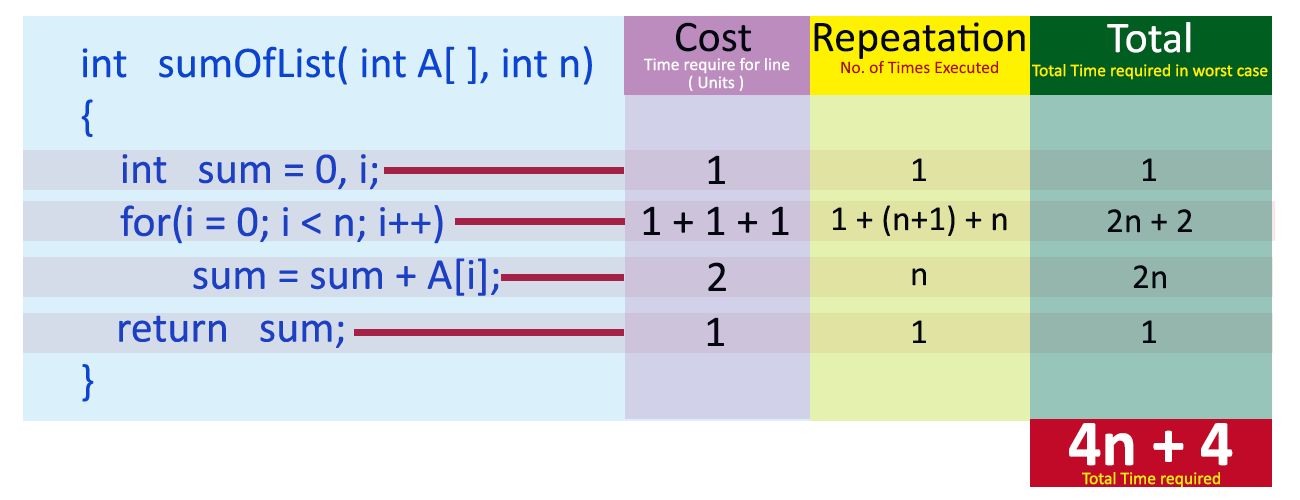The perfect place for easy learning...

×

# What is Time complexity?

Every algorithm requires some amount of computer time to execute its instruction to perform the task. This computer time required is called time complexity.
The time complexity of an algorithm can be defined as follows...

The time complexity of an algorithm is the total amount of time required by an algorithm to complete its execution.

Generally, the running time of an algorithm depends upon the following...

1. Whether it is running on Single processor machine or Multi processor machine.
2. Whether it is a 32 bit machine or 64 bit machine.
3. Read and Write speed of the machine.
4. The amount of time required by an algorithm to perform Arithmetic operations, logical operations, return value and assignment operations etc.,
5. Input data

Note - When we calculate time complexity of an algorithm, we consider only input data and ignore the remaining things, as they are machine dependent. We check only, how our program is behaving for the different input values to perform all the operations like Arithmetic, Logical, Return value and Assignment etc.,

Calculating Time Complexity of an algorithm based on the system configuration is a very difficult task because the configuration changes from one system to another system. To solve this problem, we must assume a model machine with a specific configuration. So that, we can able to calculate generalized time complexity according to that model machine.

To calculate the time complexity of an algorithm, we need to define a model machine. Let us assume a machine with following configuration...

1. It is a Single processor machine
2. It is a 32 bit Operating System machine
3. It performs sequential execution
4. It requires 1 unit of time for Arithmetic and Logical operations
5. It requires 1 unit of time for Assignment and Return value
6. It requires 1 unit of time for Read and Write operations

Now, we calculate the time complexity of following example code by using the above-defined model machine...

Consider the following piece of code...

# Example 1

``````int sum(int a, int b)
{
return a+b;
}```
```

In the above sample code, it requires 1 unit of time to calculate a+b and 1 unit of time to return the value. That means, totally it takes 2 units of time to complete its execution. And it does not change based on the input values of a and b. That means for all input values, it requires the same amount of time i.e. 2 units.

If any program requires a fixed amount of time for all input values then its time complexity is said to be Constant Time Complexity.

Consider the following piece of code...

# Example 2

``````int sum(int A[], int n)
{
int sum = 0, i;
for(i = 0; i < n; i++)
sum = sum + A[i];
return sum;
}```
```

For the above code, time complexity can be calculated as follows...In above calculation
Cost is the amount of computer time required for a single operation in each line.
Repeatation is the amount of computer time required by each operation for all its repeatations.
Total is the amount of computer time required by each operation to execute.
So above code requires '4n+4' Units of computer time to complete the task. Here the exact time is not fixed. And it changes based on the n value. If we increase the n value then the time required also increases linearly.

Totally it takes '4n+4' units of time to complete its execution and it is Linear Time Complexity.

If the amount of time required by an algorithm is increased with the increase of input value then that time complexity is said to be Linear Time Complexity.